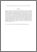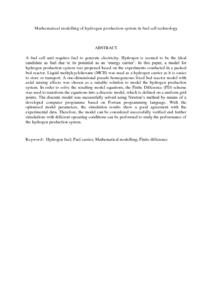# Mathematical modelling of hydrogen production system in fuel cell technology

## Citation

Murat, Muhamad Nazri and Salit, Mohd Sapuan (2008) Mathematical modelling of hydrogen production system in fuel cell technology. International Journal of Chemical Sciences, 6 (3). pp. 1385-1398. ISSN 0972-768X

## Abstract

A fuel cell unit requires fuel to generate electricity. Hydrogen is seemed to be the ideal candidate as fuel due to its potential as an ‘energy carrier’. In this paper, a model for hydrogen production system was proposed based on the experiments conducted in a packed bed reactor. Liquid methylcyclohexane (MCH) was used as a hydrogen carrier as it is easier to store or transport. A one-dimensional pseudo homogeneous fixed bed reactor model with axial mixing effects was chosen as a suitable solution to model the hydrogen production system. In order to solve the resulting model equations, the Finite Difference (FD) scheme was used to transform the equations into a discrete model, which is defined on a uniform grid points. The discrete model was successfully solved using Newton’s method by means of a developed computer programme based on Fortran programming language. With the optimised model parameters, the simulation results show a good agreement with the experimental data. Therefore, the model can be considered successfully verified and further simulations with different operating conditions can be performed to study the performance of the hydrogen production system.Preview
PDF (Abstract)
Mathematical modelling of hydrogen production system in fuel cell technology.pdfView Item